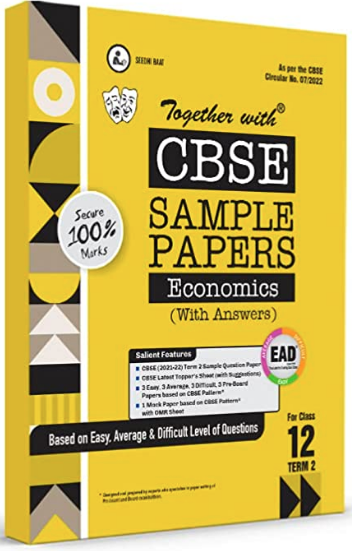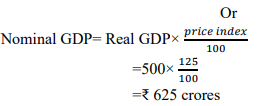# Estimate the value of Nominal Gross Domestic Product for a hypothetical economy, the value of Real Gross Domestic Product and Price Index are given as respectively.

CBSE Sample Question Paper, Class 12 ECONOMICS Term 2 Question - Estimate the value of Nominal Gross Domestic Product for a hypothetical economy, the value of Real Gross Domestic Product and Price Index are given as respectively.## Estimate the value of Nominal Gross Domestic Product for a hypothetical economy, the value of Real Gross Domestic Product and Price Index are given as respectively.